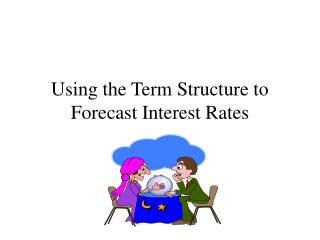DownloadDownload PresentationUsing the Term Structure to Forecast Interest Rates

# Using the Term Structure to Forecast Interest Rates

Download Presentation## Using the Term Structure to Forecast Interest Rates

- - - - - - - - - - - - - - - - - - - - - - - - - - - E N D - - - - - - - - - - - - - - - - - - - - - - - - - - -
##### Presentation Transcript

1. Using the Term Structure to Forecast Interest Rates

2. Interest Rate Forecasts • Interest rate forecasts are needed when managers of financial institutions have to set interest rates on loans that are promised to customers in the future. • We also might want to find the implied forward rate on a bond originating in the future. • Specific forecasts of the implied forward interest rate can be generated using the term structure.

3. Expectations Theory • According to the expectations theory, the expected return over two periods from investing \$1 in a two period bond must equal the expected return from investing \$1 in two one period bonds. • (1 + i2t)(1 + i2t) -1 = (1 + it)(1 + iet+1) - 1

4. The Forward Rate • (1 + i2t)(1 + i2t) -1 = (1 + it)(1 + iet+1) - 1 • (1 + i2t)2 - 1 = (1 + it)(1 + iet+1) - 1 • Solve for iet+1, the forward rate. • Add + 1 to both sides and divide by (1 + it) • 1 + iet+1 = (1 + i2t)2 / (1 + it) • Subtract + 1 from both sides • iet+1 = (1 + i2t)2 (1 + it) 1

5. Liquidity Premium • According to the liquidity premium hypothesis, investors prefer to hold short-term rather than long-term bonds. • Therefore, long-term rates include a liquidity premium to compensate the investor for accepting more risk.

6. Adjusted Forward Rate Forecast • To allow for liquidity premiums in our formula, we subtract it out. • iet+1 = (1 + i2t - l2t)2 (1 + it) 1

7. Example • Would you be willing to make a one year loan at an interest rate of 8% one year from now? To make a profit, you need to charge one percentage point more than the expected interest rate on a Treasury bond with the same maturity. • The liquidity premium is 0.4%, the one year Treasury rate is 6%, and the 2 year Treasury rate is 7%.

8. Solution • iet+1 = (1 + i2t - l2t)2 (1 + it) • iet+1 = (1 + 0.07 - 0.004)2 (1 + 0.06) • iet+1 = 7.2% • You would reject the loan. 1 1